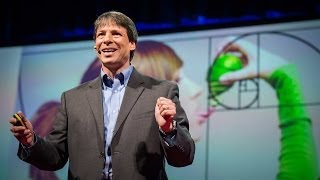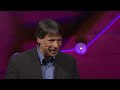# The magic of Fibonacci numbers | Arthur Benjamin

3M+ views   |   62K+ likes   |   2K+ dislikes   |
06:25   |   Nov 08, 2013

### Thumbs### Transcription

• So why do we learn mathematics?
• Essentially, for three reasons:
• calculation,
• application,
• and last, and unfortunately least
• in terms of the time we give it,
• inspiration.
• Mathematics is the science of patterns,
• and we study it to learn how to think logically,
• critically and creatively,
• but too much of the mathematics that we learn in school
• is not effectively motivated,
• and when our students ask,
• "Why are we learning this?"
• then they often hear that they'll need it
• in an upcoming math class or on a future test.
• But wouldn't it be great
• if every once in a while we did mathematics
• simply because it was fun or beautiful
• or because it excited the mind?
• Now, I know many people have not
• had the opportunity to see how this can happen,
• so let me give you a quick example
• with my favorite collection of numbers,
• the Fibonacci numbers. (Applause)
• Yeah! I already have Fibonacci fans here.
• That's great.
• Now these numbers can be appreciated
• in many different ways.
• From the standpoint of calculation,
• they're as easy to understand
• as one plus one, which is two.
• Then one plus two is three,
• two plus three is five, three plus five is eight,
• and so on.
• Indeed, the person we call Fibonacci
• was actually named Leonardo of Pisa,
• and these numbers appear in his book "Liber Abaci,"
• which taught the Western world
• the methods of arithmetic that we use today.
• In terms of applications,
• Fibonacci numbers appear in nature
• surprisingly often.
• The number of petals on a flower
• is typically a Fibonacci number,
• or the number of spirals on a sunflower
• or a pineapple
• tends to be a Fibonacci number as well.
• In fact, there are many more applications of Fibonacci numbers,
• but what I find most inspirational about them
• are the beautiful number patterns they display.
• Let me show you one of my favorites.
• Suppose you like to square numbers,
• and frankly, who doesn't? (Laughter)
• Let's look at the squares
• of the first few Fibonacci numbers.
• So one squared is one,
• two squared is four, three squared is nine,
• five squared is 25, and so on.
• Now, it's no surprise
• that when you add consecutive Fibonacci numbers,
• you get the next Fibonacci number. Right?
• That's how they're created.
• But you wouldn't expect anything special
• to happen when you add the squares together.
• But check this out.
• One plus one gives us two,
• and one plus four gives us five.
• And four plus nine is 13,
• nine plus 25 is 34,
• and yes, the pattern continues.
• In fact, here's another one.
• Suppose you wanted to look at
• adding the squares of the first few Fibonacci numbers.
• Let's see what we get there.
• So one plus one plus four is six.
• Add nine to that, we get 15.
• Add 25, we get 40.
• Add 64, we get 104.
• Now look at those numbers.
• Those are not Fibonacci numbers,
• but if you look at them closely,
• you'll see the Fibonacci numbers
• buried inside of them.
• Do you see it? I'll show it to you.
• Six is two times three, 15 is three times five,
• 40 is five times eight,
• two, three, five, eight, who do we appreciate?
• (Laughter)
• Fibonacci! Of course.
• Now, as much fun as it is to discover these patterns,
• it's even more satisfying to understand
• why they are true.
• Let's look at that last equation.
• Why should the squares of one, one, two, three, five and eight
• add up to eight times 13?
• I'll show you by drawing a simple picture.
• and next to that put another one-by-one square.
• Together, they form a one-by-two rectangle.
• Beneath that, I'll put a two-by-two square,
• and next to that, a three-by-three square,
• beneath that, a five-by-five square,
• and then an eight-by-eight square,
• creating one giant rectangle, right?
• Now let me ask you a simple question:
• what is the area of the rectangle?
• Well, on the one hand,
• it's the sum of the areas
• of the squares inside it, right?
• Just as we created it.
• It's one squared plus one squared
• plus two squared plus three squared
• plus five squared plus eight squared. Right?
• That's the area.
• On the other hand, because it's a rectangle,
• the area is equal to its height times its base,
• and the height is clearly eight,
• and the base is five plus eight,
• which is the next Fibonacci number, 13. Right?
• So the area is also eight times 13.
• Since we've correctly calculated the area
• two different ways,
• they have to be the same number,
• and that's why the squares of one, one, two, three, five and eight
• add up to eight times 13.
• Now, if we continue this process,
• we'll generate rectangles of the form 13 by 21,
• 21 by 34, and so on.
• Now check this out.
• If you divide 13 by eight,
• you get 1.625.
• And if you divide the larger number by the smaller number,
• then these ratios get closer and closer
• known to many people as the Golden Ratio,
• a number which has fascinated mathematicians,
• scientists and artists for centuries.
• Now, I show all this to you because,
• like so much of mathematics,
• there's a beautiful side to it
• that I fear does not get enough attention
• in our schools.
• We spend lots of time learning about calculation,
• but let's not forget about application,
• including, perhaps, the most important application of all,
• learning how to think.
• If I could summarize this in one sentence,
• it would be this:
• Mathematics is not just solving for x,
• it's also figuring out why.
• Thank you very much.
• (Applause)

### Description

Math is logical, functional and just ... awesome. Mathemagician Arthur Benjamin explores hidden properties of that weird and wonderful set of numbers, the Fibonacci series. (And reminds you that mathematics can be inspiring, too!)

TEDTalks is a daily video podcast of the best talks and performances from the TED Conference, where the world's leading thinkers and doers give the talk of their lives in 18 minutes (or less). Look for talks on Technology, Entertainment and Design -- plus science, business, global issues, the arts and much more.
Find closed captions and translated subtitles in many languages at http://www.ted.com/translate